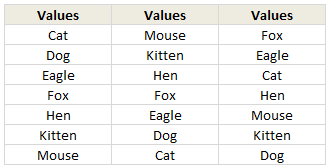# Using Array Formulas to check if a list is sorted.

Share

Today, we will learn an interesting array formula trick to test if a list is sorted or not.

During last one week, I got 2 requests from different clients for some excel related work. Both of them had one thing in common. To test whether a list is sorted or not. So I got thinking, how do I know if a list is sorted or not without visually scanning it.

Of course, the answer is in a formula.

### Let us say you have a list of values (text, numbers or mixed) like this:Assuming your values are in the range B2:B8

Write the formula `=AND(B2:B7<=B3:B8)` and press CTRL+Shift+Enter to make it an array formula.

The result will be true if the list in B2:B8  is sorted in ascending order, false otherwise.

### How does this formula work?

Very simple. Assuming your list has n values, it check first n-1 values with their next values to see if each value is less than or equal to its next value. If all of them are true, the AND formula returns TRUE. See this illustration:I have made a simple example file to illustrate this technique. Go ahead and play with it.

### How would you write formula for this?

As fun homework, go ahead and figure out an alternative formula for this. Then come back and post it comments. Let us see how many different ways we can get the same result.

Go!

### Get FREE Excel + Power BI Tips

Simple, fun and useful emails, once per week.

Learn & be awesome.

### Welcome to Chandoo.org

Thank you so much for visiting. My aim is to make you awesome in Excel & Power BI. I do this by sharing videos, tips, examples and downloads on this website. There are more than 1,000 pages with all things Excel, Power BI, Dashboards & VBA here. Go ahead and spend few minutes to be AWESOME.

Read my storyFREE Excel tips book

Chandoo is an awesome teacher
5/5

– Jason

Still on fence about Power BI? In this getting started guide, learn what is Power BI, how to get it and how to create your first report from scratch.

## Related Tips

### 25 Responses to “Using Array Formulas to check if a list is sorted.”

1.Jelle-Jeroen Lamkamp says:

This formula work for sorting A to Z but not for Z to A.

I ammended it to

=AND(AND(B2:B7AND(B2:B7>=B3:B8))

to do both

2.Shyamprasad Nayak says:

Thanks a lot!

3.Daniel Ferry says:

Very nice conjunctive truth table, Chandoo!

4.Flow says:

Jelle, I think you meant this
=OR(AND(B2:B7=B3:B8))
to sort in either ascending or descending order.

5.pfabi says:

Quite nice. I played a while with this and tuned a bit. Below formula gives the answer how the list is sorted:
=IF(AND(B1:B22=B2:B23),"Sorted Z-A","Not Sorted"))

6.pfabi says:

I don't know why but the previous formula was cut 🙁

=IF(AND(B1:B22=B2:B23),"Sorted Z-A","Not Sorted"))

7.Matt Holbrook says:

I came up with this array formula to check both ascending and descending sorts:

{=OR(AND(B2:B7=B3:B8))}

8.Matt Holbrook says:

Seems to have cut off the formula

{=OR(AND(B2:B7=B3:B8))}

9.VaraK says:

Nice One Chandoo..Best tip I read till date in 2011.

10.Jelle-Jeroen Lamkamp says:

First I meant {=AND(AND(B2:B7AND(B2:B7>=B3:B8))}
And second {=OR(AND(B2:B7=B3:B8))} doesn't work with me.
I'm running Excel 2010...

11.Jelle-Jeroen Lamkamp says:

First I meant {=AND(AND(B2:B7) AND(B2:B7>=B3:B8))}
And second {=OR(AND(B2:B7=B3:B8))} doesn’t work with me.
I’m running Excel 2010…

12.sam says:

1st new thing I learnt this year...

13.Kevin Lehrbass says:

Use formula below to see the percent of items greater than item above.
i.e. if value in D10 > value in D9 than 1, else 0. Repeat and sum the 1s and 0s.
You can change the > to \$D\$4:\$D\$9,1,0))/COUNTA(\$D\$5:\$D\$10)}

14.Kevin Lehrbass says:

Formula is: {=SUM(IF(\$D\$5:\$D\$10>\$D\$4:\$D\$9,1,0))/COUNTA(\$D\$5:\$D\$10)}

15.Karthikeyan M says:

The best tip I have ever read... Thanks....

16.Nasreen Rassulmia says:

Interesting - Thnaks Chandoo.

17.BobD says:

I am trying to use the Rank function but am getting as #Error because not all of the rows have been scored yet on my template. How do i ignore the error cells?

18.Chandoo says:

@Bob: You can suppress the errors by using IFERROR formula. Like,
=IFERROR(RANK(...),"")

If the cells are empty but have something like "N/A" in them, still this method works.

PS: IFERROR works only Excel 2007 or above.

19.Ghazanfar J says:

Cool!

20. […] Checking if a list is sorted using formulas […]

21.Jeff Weir says:

Here's a completely dynamic and non-volatile one for EXcel Tables that won't be screwed up if someone adds data or puts data above or below the Table:
{=AND(INDEX(Table1[a],2):INDEX(Table1[a],ROWS(Table1[a]))>INDEX(Table1[a],1):INDEX(Table1[a],ROWS(Table1[a])-1))}

22.indzara says:

Simple and smart trick. Thanks. This came in handy today.

23.micron1 says:

Does anyone know of a way to make this ignore blank rows?

The following didn't work:

=IF(--(A17:A26"")*--(A18:A27""),--(AND(A17:A26<=A18:A27)))

=AND(IF(--(A17:A26"")*--(A18:A27""),--(A17:A26<=A18:A27)))

Thanks!

24.micron1 says:

FYI: The following will let it ignore one blank row, and you can nest the IFs to add for more blanks in a row. Ideally it would fill down from last non-blank no matter how many blank rows.

=AND(IF(ISBLANK(A17:A27),A16:A26,A17:A27)<=IF(ISBLANK(A18:A28),A17:A27,A18:A28))

### Get FREE Excel & Power-BI Newsletter

One email per week with Excel and Power BI goodness. Join 100,000+ others and get it free.